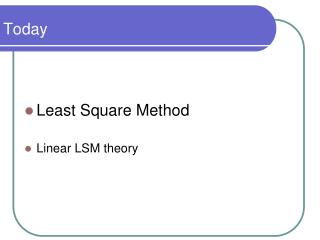DownloadDownload PresentationToday

# Today

Download Presentation## Today

- - - - - - - - - - - - - - - - - - - - - - - - - - - E N D - - - - - - - - - - - - - - - - - - - - - - - - - - -
##### Presentation Transcript

1. Today • Least Square Method • Linear LSM theory

2. Least Squares method Hypothesis: • m experimental data points (couples xj-yj) • n-th grade model given from the summation ofn functions φof x Objective: • Find the set of α parameters minimizing the deviation between the experimental values and the ones foreseen by the model, in order to have a model to estimate y, given x generic function of x

3. Least Squares method Terms to minimize:the square of the deviation between experimental data and the ones foreseen by the model Deviation of the j-th couple

4. Least Squares method the term to minimize is a positive function of α, therefore, to find a set of α for the which this function has a minimum, we can look for a unique set of α for the which all the partial derivatives are null (point of minimum)

5. Least Squares method to simplify the formulation we define φi as a column vector in the which each j-th element is the result of the φi function applied to the experimental point xj. grouping togheter the n φi columns we have matrix B of m row and n columns

6. Least Squares method if we define the column vector d as the set of all the yj. experimental points and the column vector α as the set of all the parameters, equation (1) becomes: Please Note: OUR TASK IS TO FIND α GIVEN B and d

7. Least Squares method if matrix Bwereorthogonal the product BTB would give a diagonal matrix, filled up with the norms of its basis, therefore we couldwrite we can however operate a basis transformation as to make B orthogonal, allowing us to compute α but Bis notorthogonal

8. Least Squares method to orthogonalize we use an iterative procedure:-the first new base is the same as the old-each further base is the same as the old, MINUS the dot product between the old base and the newfound basis (this is done to get rid of the interdependencies) in the new system we have Gram-Schmidt orthogonalization

9. Least Squares method following the Gram-Schmidt orthogonalization we used an iterative procedure: introducing a further term βi,p we can rewrite the direct Gram-Schmidt orthogonalization as: This is not αi!!

10. Least Squares method using an inverse iterative procedure we can trace back the original α which we sought for: This is not αi!!

11. Least Squares method First grade linear systems • Model • Orthogonalization • Orth. Model parameters • Orthogonal model

12. Least Squares method First grade linear systems • Model • Orthogonal model • Model parameters

13. Exercise 6: Linear LS • A rubber to metal device has been tested in order to assess its longitudinal stiffness obtaining the following results:

14. Exercise 7: Linear LS (2nd grade) • A bullet is shot at a unknown angle and then its distance (along the firing axis) is measured using an high-speed camera. Its initial velocity has to be determined, as well as its deceleration value.